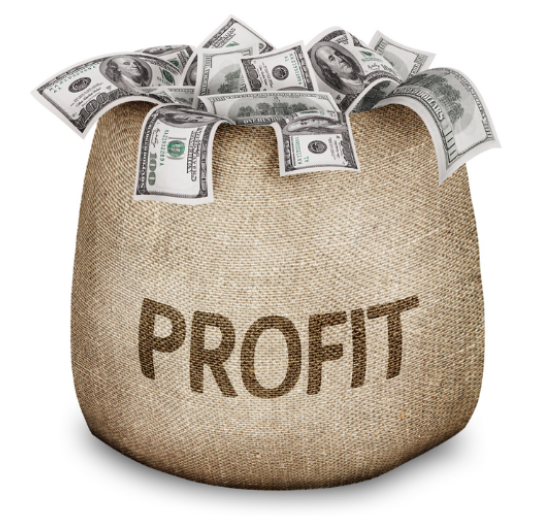# Applications of Calculus: Maximizing ProfitBusinesses wish to maximize their profit, the amount of money they get to keep once they’ve sold products and paid off the cost of producing those products. To calculate the product, you start with the total revenue (the total amount of money the business makes from selling products), and then subtract the total costs (the total amount of money the business spends on producing products).

The total revenue and total cost will depend on how many units of product a business produces. If they sell too few units, they may be able to command a high price for their product, but the high price will be offset by a high cost of producing those few units. On the other hand, if they produce too many units, scaling up their production process and making it more efficient may lead to lower costs per unit, but low costs will be offset by an even lower market price. To maximize profit, a business wants to produce just the right number of units.

If we know what the revenue and costs are of producing any number $x$ of units, then we can use calculus to figure out what number of units to produce.

Let $R(x)$ be the total revenue of producing $x$ units, and $C(x)$ be the total cost of producing $x$ units. Then the profit $P(x)$ of producing $x$ units is given by

\begin{align*} P(x) = R(x) - C(x). \end{align*}

We can find the maximum profit by taking the derivative and setting it equal to zero:

\begin{align*} 0 &= P'(x) \\[5pt] 0 &= R'(x) - C'(x) \\[5pt] C'(x) &= R'(x) \end{align*}

$C’(x)$ is the marginal cost, the cost of producing another unit, and $R’(x)$ is the marginal revenue, the money we make from selling another unit. Thus, the maximum profit occurs when marginal cost equals marginal revenue, when the cost of producing another unit equals the money we can make by selling another unit. Intuitively, that is the break-even point: if we sell another unit for the same amount of money it takes to produce it, the total profit is unaffected.

Tags: